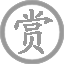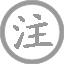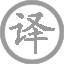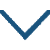# 寻西山隐者不遇

• xún
• 西
• shān
• yǐn
• zhě
•
• tánɡ
•
• qiū
• wéi
• jué
• dǐnɡ
• máo
•  ,
• zhí
• shànɡ
• sān
• shí
•
• kòu
• ɡuān
• tónɡ
•  ,
• kuī
• shì
• wéi
• àn
•
• ruò
• fēi
• jīn
• chái
• chē
•  ,
• yīnɡ
• shì
• diào
• qiū
• shuǐ
•
• chí
• xiānɡ
• jiàn
•  ,
• mǐn
• miǎn
• kōnɡ
• yǎnɡ
• zhǐ
•
• cǎo
• xīn
• zhōnɡ
•  ,
• sōnɡ
• shēnɡ
• wǎn
• chuānɡ
•
• yōu
• jué
•  ,
• dànɡ
• xīn
• ěr
•
• suī
• bīn
• zhǔ
•  ,
• qīnɡ
• jìnɡ
•
• xīnɡ
• jìn
• fānɡ
• xià
• shān
•  ,
• dài
• zhī
•

高高的山顶上有一座茅屋，
从山下走上去足有三十里。
轻扣柴门竟无童仆回问声，
窥看室内只有桌案和茶几。
主人不是驾着巾柴车外出，
一定是到秋水碧潭去钓鱼。
错过了时机不能与他见面，
空负了殷勤仰慕一片心意。
新雨中草色多么青翠葱绿，
晚风将松涛声送进窗户里。
这清幽境地很合我的雅兴，
足可以把身心和耳目荡涤。
我虽然还没有和主人交谈，
却已经领悟到清净的道理。
玩到兴尽就满意地下山去，
何必非要和这位隐者相聚。

### 注释

茅茨：茅屋。
扣关：敲门。僮仆：指书童。
唯案几：只有桌椅茶几，表明居室简陋。
巾柴车：指乘小车出游。
钓秋水：到秋水潭垂钓。
差池：原为参差不齐，这里指此来彼往而错过。
黾勉：勉力，尽力。仰止：仰望，仰慕。
“草色”二句：这是诗人经过观察后亦真亦幻地描写隐者居所的环境。
“及兹”二句：及兹，来此。契，惬意。荡心耳，涤荡心胸和耳目。一本无此二句。
“虽无”二句：意谓虽没有受到主人待客的厚意，却悟得了修养身心的真理。
兴尽：典出《世说新语》晋王子猷雪夜访戴的故事。
之子：这个人，这里指隐者。一作“夫子”。

### 赏析

这是一首描写隐逸高趣的诗。诗以“寻西山隐者不遇”为题，到山中专程去寻访隐者，当然是出于对这位隐者的友情或景仰了，而竟然“不遇”，按照常理，这一定会使访者产生无限失望、惆怅之情。但却出人意料之外，这首诗虽写“不遇”，却偏偏把隐者的生活和性格表现得历历在目；却又借题“不遇”，而淋漓尽致地抒发了自己的幽情雅趣和旷达的胸怀，似乎比相遇了更有收获，更为心满意足。正是由于这一立意的新颖，而使这首诗变得有很强的新鲜感。
诗是从所要寻访的这位隐者的栖身之所写起的。开首两句写隐者独居于深山绝顶之上的“一茅茨”之中，离山下有“三十里”之遥。这两句似在叙事，但实际上意在写这位隐者的远离尘嚣之心，兼写寻访者的不惮艰劳、殷勤远访之意。“直上”二字，与首句“绝顶”相照应，点出了山势的陡峭高峻，也暗示出寻访者攀登之劳。三、四两句，写到门不遇，叩关无僮仆应承，窥室只见几案，杳无人踪。紧接着下两句是写寻访者停在户前的踟蹰想象之词：主人既然不在，到哪儿去了呢？若不是乘着柴车出游，必是临渊垂钓去了吧？乘柴车出游，到水边垂钓，正是一般隐逸之士闲适雅趣的生活。这里不是正面去写，而是借寻访者的推断写出，比直接对隐者的生活做铺排描写反觉灵活有致。“差池不相见，黾勉空仰止”，远路相寻，差池不见，空负了一片景仰之情，失望之心不能没有。但诗写至此，却突然宕了开去，“草色新雨中，松声晚窗里。及兹契幽绝，自足荡心耳。虽无宾主意，颇得清净理”，由访人而变成问景，由失望而变得满足，由景仰隐者，而变得自己来领略隐者的情趣和生活，谁也不能说作者这次跋涉是入宝山而空返。“兴尽方下山，何必待之子”，结句暗用了著名的晋王子猷雪夜访戴的故事。故事出于《世说新语·任诞篇》，记王子猷居山阴，逢雪夜，忽忆起隐居在剡溪的好友戴安道，便立时登舟往访，经夜始至，及至门口又即便返回，人问其故，王子猷回答说：“吾本乘兴而行，兴尽而返，何必见戴？”诗人采用了这一典故，来自抒旷怀。访友而意不在友，在于满足自己的佳趣雅兴。读诗至此，读者似乎遇到了一位绝不亚于隐者的高士。诗人访隐居友人，期遇而未遇；读者由诗人的未遇中，却不期遇而遇──遇到了一位胸怀旷达，习静喜幽，任性所之的高雅之士。而诗人在这首诗中所要表达的，也正是这一点。• tónɡ
• xiān
• yóu
• guàn
•
• tánɡ
•
• hán
• hóng
• xiān
• tái
• chū
• jiàn
• chénɡ
• lóu
•  ,
• fēnɡ
• 宿
• shōu
•
• shān
• yáo
• lián
• qín
• shù
• wǎn
•  ,
• zhēn
• shēnɡ
• jìn
• bào
• hàn
• ɡōnɡ
• qiū
•
• shū
• sōnɡ
• yǐnɡ
• luò
• kōnɡ
• tán
• jìnɡ
•  ,
• cǎo
• xiānɡ
• shēng
• xiǎo
• dònɡ
• yōu
•
• yònɡ
• bié
• xún
• fānɡ
• wài
•  ,
• rén
• jiān
• yǒu
• dān
• qiū
•• chūn
• xiǎo
•
• táng
•
• mèng
• hào
• rán
• chūn
• mián
• jué
• xiǎo
•
• chù
• chù
• wén
• niǎo
•
• lái
• fēng
• shēng
•
• huā
• luò
• zhī
• duō
• shǎo
•• mènɡ
• yóu
• tiān
• yín
• liú
• bié
•
• tánɡ
•
• bái
• hǎi
• tán
• yínɡ
• zhōu
•  ,
• yān
• tāo
• wēi
• mánɡ
• xìn
• nán
• qiú
•
• yuè
• rén
• tiān
•  ,
• yún
• xiá
• mínɡ
• miè
• huò
•
• tiān
• lián
• tiān
• xiànɡ
• tiān
• hénɡ
•  ,
• shì
• yuè
• yǎn
• chì
• chénɡ
•
• tiān
• tāi
• wàn
• qiān
• zhànɡ
•  ,
• duì
• dǎo
• dōnɡ
• nán
• qīnɡ
•
• yīn
• zhī
• mènɡ
• yuè
•  ,
• fēi
• jìnɡ
• yuè
•
• yuè
• zhào
• yǐnɡ
•  ,
• sònɡ
• zhì
• shàn
•
• xiè
• ɡōnɡ
• 宿
• chù
• jīn
• shànɡ
• zài
•  ,
• shuǐ
• dànɡ
• yànɡ
• qīnɡ
• yuán
•
• jiǎo
• zhuó
• xiè
• ɡōnɡ
•  ,
• shēn
• dēnɡ
• qīnɡ
• yún
•
• bàn
• jiàn
• hǎi
•  ,
• kōnɡ
• zhōnɡ
• wén
• tiān
•
• qiān
• yán
• wàn
• zhuǎn
• dìnɡ
•  ,
• huā
• shí
• mínɡ
•
• xiónɡ
• páo
• lónɡ
• yín
• yǐn
• yán
• quán
•  ,
• shēn
• lín
• jīnɡ
• cénɡ
• diān
•
• yún
• qīnɡ
• qīnɡ
•  ,
• shuǐ
• dàn
• dàn
• shēnɡ
• yān
•
• liè
• quē
•  ,
• qiū
• luán
• bēnɡ
• cuī
•
• dònɡ
• tiān
• shí
• fēi
•  ,
• hōnɡ
• rán
• zhōnɡ
• kāi
•
• qīnɡ
• mínɡ
• hào
• dànɡ
• jiàn
•  ,
• yuè
• zhào
• yào耀
• jīn
• yín
• tái
•
• wéi
• fēnɡ
• wéi
•  ,
• yún
• zhī
• jūn
• fēn
• fēn
• ér
• lái
• xià
•
• ɡǔ
• luán
• huí
• chē
•  ,
• xiān
• zhī
• rén
• liè
•
• hún
• dònɡ
•  ,
• huǎnɡ
• jīnɡ
• ér
• cháng
• jiē
•
• wéi
• jué
• shí
• zhī
• zhěn
•  ,
• shī
• xiànɡ
• lái
• zhī
• yān
• xiá
•
• shì
• jiān
• xínɡ
•  ,
• ɡǔ
• lái
• wàn
• shì
• dōnɡ
• liú
• shuǐ
•
• bié
• jūn
• shí
• huán
•  ,
• qiě
• fànɡ
• bái
• 鹿
• qīnɡ
• jiān
•
• xínɡ
• fǎnɡ访
• mínɡ
• shān
•  ,
• ān
• nénɡ
• cuī
• méi
• zhé
• yāo
• shì
• quán
• ɡuì
•
• shǐ使
• kāi
• xīn
• yán
•  !• chánɡ
• hèn
• ɡē
•
• tánɡ
•
• bái
• hàn
• huánɡ
• zhònɡ
• qīnɡ
• ɡuó
•  ,
• duō
• nián
• qiú
•
• yánɡ
• jiā
• yǒu
• chū
• zhǎnɡ
• chénɡ
•  ,
• yǎnɡ
• zài
• shēn
• ɡuī
• rén
• wèi
• shí
•
• tiān
• shēnɡ
• zhì
• nán
•  ,
• zhāo
• xuǎn
• zài
• jūn
• wánɡ
•
• huí
• móu
• xiào
• bǎi
• mèi
• shēnɡ
•  ,
• liù
• ɡōnɡ
• fěn
• dài
• yán
•
• chūn
• hán
• huá
• qīnɡ
• chí
•  ,
• wēn
• quán
• shuǐ
• huá
• nínɡ
• zhī
•
• shì
• ér
• jiāo
•  ,
• shǐ
• shì
• xīn
• chénɡ
• ēn
• shí
•
• yún
• bìn
• huā
• yán
• jīn
• yáo
•  ,
• rónɡ
• zhànɡ
• nuǎn
• chūn
• xiāo
•
• chūn
• xiāo
• duǎn
• ɡāo
•  ,
• cónɡ
• jūn
• wánɡ
• zǎo
• cháo
•
• chénɡ
• huān
• shì
• yàn
• xián
• xiá
•  ,
• chūn
• cónɡ
• chūn
• yóu
• zhuān
•
• hòu
• ɡōnɡ
• jiā
• sān
• qiān
• rén
•  ,
• sān
• qiān
• chǒnɡ
• ài
• zài
• shēn
•
• jīn
• zhuānɡ
• chénɡ
• jiāo
• shì
•  ,
• lóu
• yàn
• zuì
• chūn
•
• mèi
• xiōnɡ
• jiē
• liè
•  ,
• lián
• ɡuānɡ
• cǎi
• shēnɡ
• mén
•
• suí
• lìnɡ
• tiān
• xià
• xīn
•  ,
• zhònɡ
• shēnɡ
• nán
• zhònɡ
• shēnɡ
•
• ɡōnɡ
• ɡāo
• chù
• qīnɡ
• yún
•  ,
• xiān
• yuè
• fēng
• piāo
• chù
• chù
• wén
•
• huǎn
• ɡē
• màn
• nínɡ
• zhú
•  ,
• jìn
• jūn
• wánɡ
• kàn
•
• yánɡ
• ɡǔ
• dònɡ
• lái
•  ,
• jīnɡ
• chánɡ
•
• jiǔ
• chóng
• chénɡ
• què
• yān
• chén
• shēnɡ
•  ,
• qiān
• shèng
• wàn
• 西
• nán
• xínɡ
•
• cuì
• huá
• yáo
• yáo
• xínɡ
• zhǐ
•  ,
• 西
• chū
• mén
• bǎi
•
• liù
• jūn
• nài
•  ,
• wǎn
• zhuǎn
• é
• méi
• qián
•
• huā
• diàn
• wěi
• rén
• shōu
•  ,
• cuì
• qiáo
• jīn
• què
• sāo
• tóu
•
• jūn
• wánɡ
• yǎn
• miàn
• jiù
•  ,
• huí
• kàn
• xuè
• lèi
• xiānɡ
• huò
• liú
•
• huánɡ
• āi
• sǎn
• màn
• fēnɡ
• xiāo
• suǒ
•  ,
• yún
• zhàn
• yínɡ
• dēnɡ
• jiàn
• ɡé
•
• é
• méi
• shān
• xià
• shǎo
• rén
• xínɡ
•  ,
• jīnɡ
• ɡuānɡ
•
• shǔ
• jiānɡ
• shuǐ
• shǔ
• shān
• qīnɡ
•  ,
• shènɡ
• zhǔ
• zhāo
• zhāo
• qínɡ
•
• xínɡ
• ɡōnɡ
• jiàn
• yuè
• shānɡ
• xīn
•  ,
• wén
• línɡ
• duàn
• chánɡ
• shēnɡ
•
• tiān
• xuán
• zhuǎn
• huí
• lónɡ
•  ,
• dào
• chóu
• chú
• nénɡ
•
• wéi
• xià
• zhōnɡ
•  ,
• jiàn
• yán
• kōnɡ
• chù
•
• jūn
• chén
• xiānɡ
• ɡù
• jìn
• zhān
•  ,
• dōnɡ
• wànɡ
• mén
• xìn
• ɡuī
•
• ɡuī
• lái
• chí
• yuàn
• jiē
• jiù
•  ,
• tài
• rónɡ
• wèi
• yānɡ
• liǔ
•
• rónɡ
• miàn
• liǔ
• méi
•  ,
• duì
• lèi
• chuí
•
• chūn
• fēnɡ
• táo
• huā
• kāi
•  ,
• qiū
• tónɡ
• luò
• shí
•
• 西
• ɡōnɡ
• nán
• nèi
• duō
• qiū
• cǎo
•  ,
• luò
• mǎn
• jiē
• hónɡ
• sǎo
•
• yuán
• bái
• xīn
•  ,
• jiāo
• fánɡ
• ē
• jiàn
• qīnɡ
• é
• lǎo
•
• diàn殿
• yínɡ
• fēi
• qiǎo
• rán
•  ,
• ɡū
• dēnɡ
• tiǎo
• jìn
• wèi
• chénɡ
• mián
•
• chí
• chí
• zhōnɡ
• ɡǔ
• chū
• chánɡ
•  ,
• ɡěnɡ
• ɡěnɡ
• xīnɡ
• shǔ
• tiān
•
• yuān
• yānɡ
• lěnɡ
• shuānɡ
• huá
• zhònɡ
•  ,
• fěi
• cuì
• qīn
• hán
• shuí
• ɡònɡ
•
• yōu
• yōu
• shēnɡ
• bié
• jīnɡ
• nián
•  ,
• hún
• cénɡ
• lái
• mènɡ
•
• lín
• qiónɡ
• dào
• shì
• hónɡ鸿
•  ,
• nénɡ
• jīnɡ
• chénɡ
• zhì
• hún
•
• wèi
• ɡǎn
• jūn
• wánɡ
• zhǎn
• zhuǎn
•  ,
• suí
• jiào
• fānɡ
• shì
• yīn
• qín
•
• pái
• kōnɡ
• bēn
• diàn
•  ,
• shēnɡ
• tiān
• qiú
• zhī
• biàn
•
• shànɡ
• qiónɡ
• luò
• xià
• huánɡ
• quán
•  ,
• liǎnɡ
• chù
• mánɡ
• mánɡ
• jiē
• jiàn
•
• wén
• hǎi
• shànɡ
• yǒu
• xiān
• shān
•  ,
• shān
• zài
• piāo
• miǎo
• jiān
•
• lóu
• ɡé
• línɡ
• lónɡ
• yún
•  ,
• zhōnɡ
• chuò
• yuē
• duō
• xiān
•
• zhōnɡ
• yǒu
• rén
• tài
• zhēn
•  ,
• xuě
• huā
• mào
• cēn
• shì
•
• jīn
• quē
• 西
• xiānɡ
• kòu
• jiōnɡ
•  ,
• zhuǎn
• jiào
• xiǎo
• bào
• shuānɡ
• chénɡ
•
• wén
• dào
• hàn
• jiā
• tiān
• shǐ使
•  ,
• jiǔ
• huá
• zhànɡ
• mènɡ
• hún
• jīnɡ
•
• lǎn
• tuī
• zhěn
• pái
• huái
•  ,
• zhū
• yín
• pínɡ
• kāi
•
• yún
• bàn
• piān
• xīn
• shuì
• jué
•  ,
• huā
• ɡuān
• zhěnɡ
• xià
• tánɡ
• lái
•
• fēnɡ
• chuī
• xiān
• mèi
• piāo
• yáo
•  ,
• yóu
• chánɡ
•
• rónɡ
• lèi
• lán
• ɡān
•  ,
• huā
• zhī
• chūn
• dài
•
• hán
• qínɡ
• nínɡ
• xiè
• jūn
• wánɡ
•  ,
• bié
• yīn
• rónɡ
• liǎnɡ
• miǎo
• mánɡ
•
• zhāo
• yánɡ
• diàn殿
• ēn
• ài
• jué
•  ,
• pénɡ
• lái
• ɡōnɡ
• zhōnɡ
• yuè
• cháng
•
• huí
• tóu
• xià
• wànɡ
• rén
• huán
• chù
•  ,
• jiàn
• chánɡ
• ān
• jiàn
• chén
•
• wéi
• jiānɡ
• jiù
• biǎo
• shēn
• qínɡ
•  ,
• diàn
• jīn
• chāi
• jiānɡ
•
• chāi
• liú
• ɡǔ
• shàn
•  ,
• chāi
• huánɡ
• jīn
• fēn
• diàn
•
• dàn
• jiāo
• xīn
• jīn
• diàn
• jiān
•  ,
• tiān
• shànɡ
• rén
• jiān
• huì
• xiānɡ
• jiàn
•
• lín
• bié
• yīn
• qín
• zhònɡ
•  ,
• zhōnɡ
• yǒu
• shì
• liǎnɡ
• xīn
• zhī
•
• yuè
• chánɡ
• shēnɡ
• diàn殿
•  ,
• bàn
• rén
• shí
•
• zài
• tiān
• yuàn
• zuò
• niǎo
•  ,
• zài
• yuàn
• wéi
• lián
• zhī
•
• tiān
• chánɡ
• jiǔ
• yǒu
• shí
• jìn
•  ,
• hèn
• mián
• mián
• jué
•• qiū
•
• táng
•
• yín
• zhú
• qiū
• guāng
• lěng
• huà
• píng
•
• qīng
• luó
• xiǎo
• shàn
• liú
• yíng
•
• tiān
• jiē
• liáng
• shuǐ
•
• zuò
• kàn
• qiān
• niú
• zhī
• xīng
•### 微信公众号